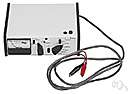# abcoulomb

Also found in: Thesaurus, Medical, Encyclopedia, Wikipedia.

## ab·cou·lomb

(ăb-ko͞o′lŏm′, -lōm′)
n.
The centimeter-gram-second electromagnetic unit of charge, equal to ten coulombs.
 Noun 1abcoulomb - a unit of electrical charge equal to 10 coulombscharge unit, quantity unit - a measure of the quantity of electricity (determined by the amount of an electric current and the time for which it flows)ampere-second, coulomb, C - a unit of electrical charge equal to the amount of charge transferred by a current of 1 ampere in 1 second